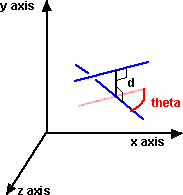# Maths - Intersections of lines

The intersection of two lines is a point. However, this only applies if the lines intersect, otherwise the intersection is null.

## In Two Dimensions

In two dimensions the lines will intersect if they are not parallel, that is, if they have a different slope.

• if the lines are the same the intersection is the whole line.
• if the lines are parallel but not the same then the intersection is null.
• if the lines are neither the same or parallel then the intersection is a point.

## In Three or more Dimensions

When lines are in 3 dimensions it is possible that the lines do not intersect, being in two different planes.We can use dual numbers to represent skew lines as explained here.

The relationship between the lines is represented by the dual number:

theta + d D

where:

• theta is the angle between the lines (in radians)
• d is the distance between the lines
• D is the dual operator

if theta=0 then the lines are parallel

if d=0 then the lines intersect

## Meet

For a wider discussion of intersection of 2D shapes see this page.New Foundations for Classical Mechanics (Fundamental Theories of Physics). This is very good on the geometric interpretation of this algebra. It has lots of insights into the mechanics of solid bodies. I still cant work out if the position, velocity, etc. of solid bodies can be represented by a 3D multivector or if 4 or 5D multivectors are required to represent translation and rotation.